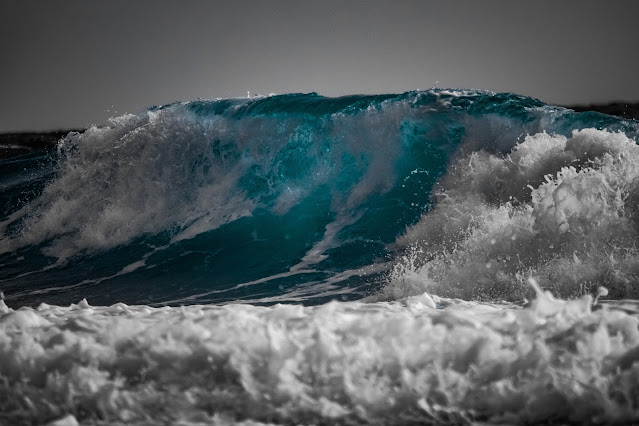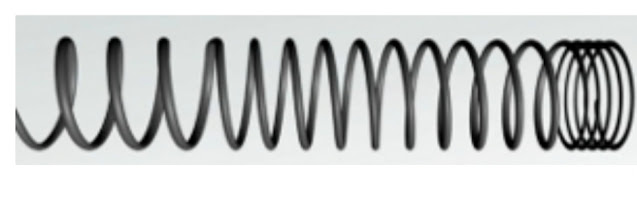## Pages

### Wave motion  wave motion is 'which propagates in all directions, from the point of generation, without actual translatory motion of par...Wave motion

wave motion is 'which propagates in all directions, from the point of generation, without actual translatory motion of particles of the medium', and the propagation of the wave is called 'wave motion'.
Wave motion is classified into three different ways they are,
• The energy transfer
• The medium of propagation,
• The dimensions in which a wave propagates energy

Table of content
• Transverse wave motion
• Longitudinal Wave motion
• physics wave motion questions and answers

## Transverse wave motion

The circular ripples produced on the surface of water expand and propagate through water. As the ripples move horizontally across the surface of water, the water particles vibrate and down. Thus, as the water waves (ripples) propagate horizontally, the particles of the medium (here water) vibrate in a transverse (perpendicular) direction to the direction of wave propagation. Such waves are known as 'transverse waves', Thus, transverse waves are defined as, 'the waves in which the particles of the medium vibrate in a direction perpendicular to the direction of wave propagation'. Ripples are the waves formed on surface of water and so these are also called surface waves.Figure 1 Transverse wave in spring

Consider a string of a certain length, with one end attached to a fixed support. Hold the other free end of the string in hand, stretch and vibrate it in a perpendicular direction to the length of the string. A wave pattern is observed in the string as shown in the Fig. 1.

The rest position or mean position of the string is shown by a dotted line in the figure. As the wave propagates from left to right, the particles of the string vibrate up and down, thus forming a transverse wave in the string. At a given moment there are some positions in the wave where the vibrating particles have maximum displacement in the upward direction from the mean position.

These positions are known as 'crests' and are shown by 'C' in the Fig. 1. At the same moment, there are some positions in the wave, where the vibrating particles have maxinmum displacement in the downward direction from the mean position. These positions are known as 'troughs' and are shown by 'T' in the Fig. 1. This maximum displacement of the vibrating particle from the mean position, either upward or downward, is known as 'amplitude'. The wave pattern between two successive crests or troughs is fixed for a given wave form and the whole wave can be obtained by repetition of this patter. So, the distance between two successive crests' or between two successive 'troughs' is constant for a given wave and is known as 'wavelength', a physical quantity with which a wave is characterized. It is denoted by the Greek letter (lambda). The time taken to produce one complete wave is known as "time period', denoted by 'T'and the number of complete waves produced in one second of time is known as 'frequency', denoted by 'n' and measured in 'hertz' (Hz).

## Longitudinal Wave motionFigure 2 a long spring

Consider a long spring placed on a table and fixed at one end as shown in Fig. 2. with the other end remaining free. If we give a gentle pull to the free end and leave it, the spring executes oscillations along its length. The rings of the spring will oscillate to and fro about their mean positions or rest positions and the pull given at the free end of the spring travels to the other end.Figure 3 formation of longitudinal wave

As the rings of the spring vibrate to and fro , we find that at any given moment , in some regions in the spring the distance between two adjacent rings is less than the distance between them before the disturbance was started . Such regions are known as ' compressions and are denoted by ' C'in Fig . 3.

Similarly , at the same moment there are some regions in the spring , where the distance between two adjacent rings is larger than the distance between them when they were at the mean position . Such regions in the spring are known as ' rarefactions and are denoted by R in Fig . 3. As the spring vibrates , a series of compressions and rarefactions propagate from one end to the other end of the spring and so energy is propagated from one end to another without translatory motion of the particles . Thus , a wave is formed . In this wave , the particles of the medium ( here the rings ) vibrate in a direction parallel to the direction of the wave propagation . Such a wave is known as a " longitudinal wave ' . Thus , a longitudinal wave is defined as a wave in which the particles of the medium vibrate in a direction parallel to the direction of wave propagation ' . So , longitudinal waves propagate in a medium in the form of compressions and rarefactions . Compressions are also known as " condensations ' . Sound wave travelling in air or in any gas is an example of longitudinal wave propagation .

In the case of longitudinal waves , the distance between two successive compressions or the distance between two successive rarefactions is known as ' wavelength ' and the time interval between two successive compressions or the time interval between two successive rarefactions at a given point is known as time period of the wave propagation . The number of compressions or rarefactions produced in unit time is known as ' frequency ' .

## physics wave motion questions and answers

1. Transverse mechanical waves can propagate
Answer- in a metal but not in a gas.

2. A sound wave travels 60 m in the time taken to pro-duce 50 waves. Find the wave in centimeters.
Answer- (i) One wave is equal to one wavelength.
(ii) 120 cm